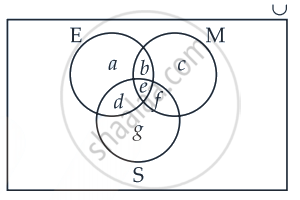# Out of 100 students; 15 passed in English, 12 passed in Mathematics, 8 in Science, 6 in English and Mathematics, 7 in Mathematics and Science; 4 in English and Science; 4 in all the three. Find - Mathematics

Diagram
Sum

Out of 100 students; 15 passed in English, 12 passed in Mathematics, 8 in Science, 6 in English and Mathematics, 7 in Mathematics and Science; 4 in English and Science; 4 in all the three. Find how many passed in Mathematics only

#### Solution

Let the number of students passed in Mathematics M, E be in English and S be in Science.

Then n(U) = 100

n(M) = 12

n(E) = 15

n(S) = 8

n(E ∩ M) = 6

n(M ∩ S) = 7

n(E ∩ S) = 4

And n(E ∩ M ∩ S) = 4

Let us draw a Venn diagram.According to the Venn diagram, we get

a + b + d + e = 15   ......(i)

b + c + e + f = 12  ......(ii)

d + e + f + g = 8  .....(iii)

n(E ∩ M) = 6

∴ b + e = 6  ......(iv)

n(M ∩ S) = 7

∴ e + f = 7  ......(v)

n(E ∩ S) = 4

∴ d + e = 4  ......(vi)

And n(E ∩ M ∩ S) = 4

∴ e = 4  ......(vii)

From equation (iv) and (vii)

We get b + 4 = 6

∴ b = 2

From equation (v) and (vii)

We get 4 + f = 7

∴ f = 3

From equation (vi) and (vii)

We get d + 4 = 4

∴ d = 0

From equation (i) we get

a + b + d + e = 15

⇒ a + 2 + 0 + 4 = 15

⇒ a = 9

From equation (ii)

b + c + e + f = 12

⇒ 2 + c + 4 + 3 = 12

⇒ c = 3

From equation (iii)

d + e + f + g = 8

⇒ 0 + 4 + 3 + g = 8

⇒ g = 1

∴ Number of students who passed in Mathematics only, c = 3.

Concept: Sets and Their Representations
Is there an error in this question or solution?

#### APPEARS IN

NCERT Mathematics Exemplar Class 11
Chapter 1 Sets
Exercise | Q 24.(iii) | Page 14

Share